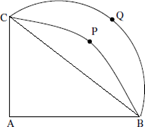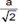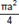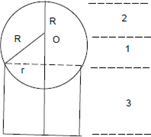# CAT 2017 – Slot 1 – Quantitative Ability – From a triangle ABC with sides of lengths 40 ft, 25 ft and 35 ft, a triangular portion GBC is cut

Q. 1:From a triangle ABC with sides of lengths 40 ft, 25 ft and 35 ft, a triangular portion GBC is cut off where G is the centroid of ABC. The area, in sq ft, of the remaining portion of triangle ABC is
A) 225√3
B) 500 / √3
C) 275 / √3
D) 250 / √3

Area of triangle = root (s(s-a)(s-b)(s-c))

S = 50

Area = 250 * root(3)

Centroid divides triangle in the ratio 2:1

Area of remaining portion of triangle: 2/3 * (Area of Triangle) = 500/root(3)

Option (B)

##### Q. 2: Let ABC be a right-angled isosceles triangle with hypotenuse BC. Let BQC be a semi-circle, away from A, with diameter BC. Let  BPC be an arc of a circle centered at A and lying between BC and BQC. If AB has length 6 cm then the area, in sq cm, of the  region enclosed by BPC and BQC isA) 9π – 18 B) 18 C) 9π D) 9Explanation:-  Let AB = a (a = 6)

CQB is a semicircle of radius∴ Area of semicircle =Area of quadrant =∴ Area of region enclosed by BPC, BQC = Area of ∆ABC = 18.

Q. 3: A solid metallic cube is melted to form five solid cubes whose volumes are in the ratio 1 : 1 : 8: 27: 27. The percentage by which the sum of the surface areas of these five cubes exceeds the surface area of the original cube is nearest to
A) 10
B) 50
C) 60
D) 20

The volumes of the 5 smaller cubes and the original big one are in the ratio 1 : 1 : 8 : 27 : 27 : 64. Therefore, the sides are in the   ratio 1 : 1 : 2 : 3 : 3 : 4 while the areas are in the ratio 1 : 1 : 4 : 9 : 9 : 16. The sum of the areas of the 5 smaller cubes is 24 parts  while that of the big cube is 16 parts. The sum is 50% greater.

Q. 4: A ball of diameter 4 cm is kept on top of a hollow cylinder standing vertically. The height of the cylinder is 3 cm, while its volume is 9 π cm3. Then the vertical distance, in cm, of the topmost point of the ball from the base of the cylinder isExplanation:-

The height of the cylinder (h) = 3 The volume = 9π

πr2h = 9π ⇒ r = √3

The radius of the ball (R) = 2

The height of O, the centre of the ball, above the line representing the top of the cylinder is say a. (a = 1)

∴ The height of the topmost point of the ball from the base of the cylinder is h + a +R = 3 + 1 + 2 = 6

Q. 5: Let ABC be a right-angled triangle with BC as the hypotenuse. Lengths of AB and AC are 15 km and 20 krn, respectively. The minimum possible time, in minutes, required to reach the hypotenuse from A at a speed of 30 km per hour is

Explanation:-  In a 3, 4, 5 triangle, the length of the altitude to the hypotenuse = 3(4)/5 = 2.4. Therefore, in a 15, 20, 25 triangle,   it is 12. This is the shortest distance from A to BC. At 60 km/hr, i.e., 1 km/min, it would take 24 min to cover 24 km.

##### Checkout Other Questions of CAT 2017 Slot 1 Paper:

Verbal Ability :              |   Q.01- Q.06  |  Q.07- Q.12  |  Q.13- Q.18  |  Q.19- Q.21  |  Q.22- Q.24  |  Q.25- Q.29  |  Q.30 – Q.34  |

Logical Reasoning :    |   Q.29 – Q.32  |

Quantitative Aptitude: |

### Free Material Area